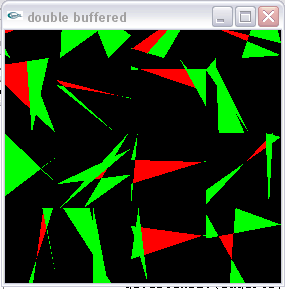Previous Entry Next Entry→

August 24, 2005

Take a dash of trip to the beach, a hefty helping of jury duty and mix with intractable probability problem and you've got a recipe for time consumption.

 The code is now to where I it sorts the random triangle vertices in increasing order of x coordinates, computes the edge coordinates and makes a very rudimentary attempt to draw the "shadow polygons" where a fourth point would not form a convex quadrilateral.  There is a constant float called "GRID" which allows for this to happen repeatedly in one plot.  With GRID set to 4, I get, for instance, 16 plots in a picture like the one shown at right.  The picture is badly cropped (I need to learn a few things about saving these pics) The original random triangle is the red one.  If it works out right, each vertex of the red triangle touches a green triangle at a single point, forming congruent vertex angles at that point.  This ideal is most clearly realized in the lower right of the 16 examples shown in the graphic at right.// Based on code from OpenGl Primer by Edward Angel
#include <GL/glut.h>
#include <math.h>
#include <iostream>
#include <ctime>
#include <cstdlib>
using namespace std;
#define NULL 0

const float GRID = 4.0;

int doubleb; //window ids //singleb,

//prototypes
void display();
void keyIt(unsigned char, int, int);
void reshapeIt(int, int);
void display();
void drawObjects(GLenum);
void sortVertices(float );
void printVertices(float );
void edgePoints(float ,float ,float,float);

////////////////////////////////////////
int main(int argc, char** argv) {
glutInit(&argc, argv);
//create a double buffered window
glutInitDisplayMode(GLUT_DOUBLE | GLUT_RGB);
doubleb = glutCreateWindow("double buffered");
//initIt();
glutDisplayFunc(display);
glutReshapeFunc(reshapeIt);
glutIdleFunc(display);
//glutMouseFunc(mouse);
//Enter event loop
glutMainLoop();
}

void display() {
glClear(GL_COLOR_BUFFER_BIT);
drawObjects(GL_RENDER);
glutSwapBuffers();
}

void drawObjects(GLenum mode) {
float t;
float edgePts;
char a;
// Draw a 10X10 grid of triangole pictures.
for(float i = 0; i < 1.0; i+=1./GRID) {
for(float j = 0; j < 1.0; j+=1./GRID) {
for(int k= 0;k<3;++k) {
t[k] = (float) rand()/(GRID*RAND_MAX)+i;
t[k] = (float) rand()/(GRID*RAND_MAX)+j;
}
sortVertices(t);
printVertices(t);
// Compute and report edgepoints for each triangle
edgePoints(t,edgePts,i,j);
glColor3f(1.0,0.0,0.0); //red
glBegin(GL_TRIANGLES);
glVertex2fv(t);
glVertex2fv(t);
glVertex2fv(t);
glEnd();
glColor3f(0.0,1.0,0.0);
glBegin(GL_TRIANGLES);
glVertex2fv(t);
glVertex2fv(edgePts);
glVertex2fv(edgePts);
glVertex2fv(t);
glVertex2fv(edgePts);
glVertex2fv(edgePts);
glVertex2fv(t);
glVertex2fv(edgePts);
glVertex2fv(edgePts);
glEnd();
cin.get(a);
}
}
}

void sortVertices(float t) {
float temp;
if(t<t) {
temp = t;
t = t;
t = temp;
temp = t;
t = t;
t = temp;
}
if(t<t) {
temp = t;
t = t;
t = temp;
temp = t;
t = t;
t = temp;
}
if(t<t) {
temp = t;
t = t;
t = temp;
temp = t;
t = t;
t = temp;
}
}

void edgePoints(float t, float edgePts, float xIndex, float yIndex) {
float x,y;

// Sort the points in increasing order of x.
// Note: this should be tightened up with a bubble sort...

// compute where triangle lines intersect perimeter of unit square
// start by computing the three slopes:

float m;
m = (t - t)
/(t - t);
m = (t - t)
/(t - t);
m = (t - t)
/(t - t);
// for each vertex, compute the other two vertices of the
// polygon whose area we want, which are where the extended
// triangle edges meet the perimeter of the unit square.
// x0,y0
int next = 0;
//for each extended edge
for(int e = 0; e < 3; ++e) {
y = t[e] + m[e]*(xIndex-t[e]);
if(yIndex<y && y<yIndex+1./GRID) {
edgePts[next] = xIndex;
edgePts[next] = y;
++next;
}
y = t[e] + m[e]*(xIndex+1./GRID-t[e]);
if(yIndex<y && y<yIndex+1./GRID) {
edgePts[next] = xIndex+1./GRID;
edgePts[next] = y;
++next;
}
x = t[e] + (yIndex-t[e])/m[e];
if(xIndex<x && x<xIndex+1./GRID) {
edgePts[next] = x;
edgePts[next] = yIndex;
++next;
}
x = t[e] +(yIndex+1./GRID-t[e])/m[e];
if(xIndex<x && x<xIndex+1./GRID) {
edgePts[next] = x;
edgePts[next] = yIndex+1./GRID;
++next;
}
}
// print out
cout << "\nThe edge points are:\n";
for(int i = 0; i < 6; ++i)
cout << edgePts[i] << " " << edgePts[i] << endl;
//cin >> x;

}

void reshapeIt(int w, int h) {
glViewport(0,0,w,h);
glMatrixMode(GL_PROJECTION);
gluOrtho2D(0.0,1.0,0.0,1.0);
glMatrixMode(GL_MODELVIEW);
}
void printVertices(float t) {
cout << "\nThe triangle has coordinates: \n";
for(int i = 0; i < 3; ++i)
cout << t[i] << " " << t[i] << endl;
}
// Both a keyboard and a mouse callback are illustrated here
/*void keyIt(unsigned char key, int x, int y) {
if(key == 'Q' || key == 'q') exit(0);
}